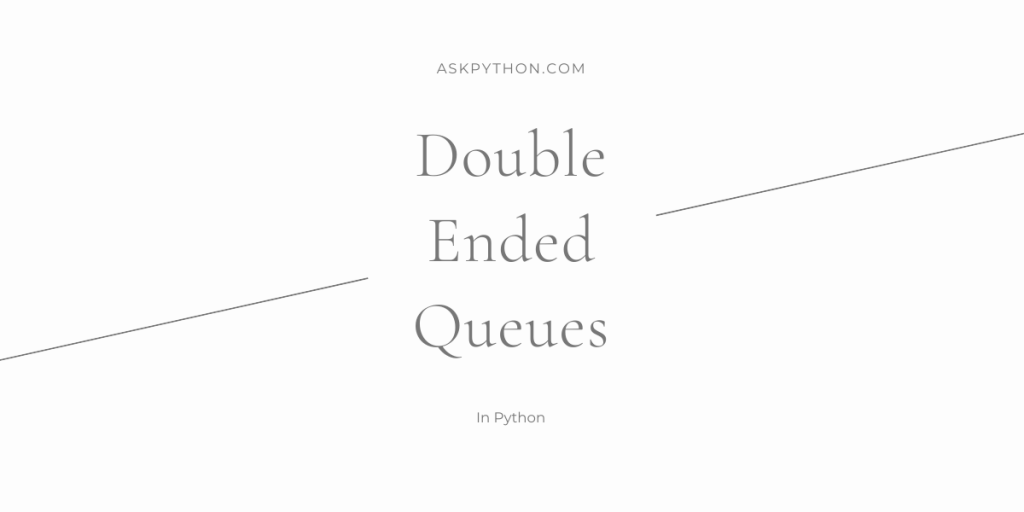# Double-Ended Queue in PythonA Double-Ended Queue is a data structure that is used to store a collection of items that are meant to be in a queue. It is an extension of the queue data structure with some additional features.

## Pre-Requisite: Queue

A queue is a data structure that is used to store a collection of items in the manner of a real-life queue. In a real queue, people usually enter from the back and exit from the front after they move through the queue. This is called a First-In-First-Out procedure.

A queue data structure is a similarly implemented list, in which all data is entered at the end of the list and all data is removed at the start of the list.

## Implementing a Double-Ended Queue in Python

In a double-ended queue, as the name suggests, data can be added and removed from the front as well as from the back, but data cannot be added or removed in the middle of the queue. Double-ended queues are also called deques.

We will now see its implementation in python. We will not be using the inbuilt `collections` package, instead, we will implement it ourselves.

## Class: Deque

```class Deque:
def __init__(self):
self.queue = []
self.count = 0

def __repr__(self):
str = ""
if self.count == 0:
str += "Double Ended Queue Empty."
return str
str += "Double Ended Queue:\n" + self.queue.__repr__()
return str

def insert_start(self, data):
if self.count == 0:
self.queue = [data,]
self.count = 1
return

self.queue.insert(0, data)
self.count += 1
return

def insert_end(self, data):
if self.count == 0:
self.queue = [data,]
self.count = 1
return

self.queue.append(data)
self.count += 1
return

def remove_start(self):
if self.count == 0:
raise ValueError("Invalid Operation")

x = self.queue.pop(0)
self.count -= 1
return x

def remove_end(self):
if self.count == 0:
raise ValueError("Invalid Operation")

x = self.queue.pop()
self.count -= 1
return x

def get(self, index):
if index >= self.count | index < 0:
raise ValueError("Index out of range.")

return self.queue[index]

def size(self):
return self.count

def display(self):
print(self)
return
```

This is the code for a double-ended queue. There’s many methods, let us discuss them one by one.

### 1. The `__init__` and `__repr__` methods

In the `__init__` method, we declare a list named `queue` that will contain the deque, and a counter to count the number of items in the list.

In the `__repr__` method, we create the string that will be used to print the double-ended queue.

### 2. The `insert_start` and `insert_end` methods

In the `insert_start` method, we simply insert the new element at index `0` of the list `queue`, and we increment the count of items in the list.

In the `insert_end` method, we simply append the new item in the list `queue`, and we increment the count of items in the list.

### 3. The `remove_start` and `remove_end` methods

In the `remove_start` method, we check if the list is empty, and if so, then we raise a `ValueError`. After that, we pop the item at index `0`, decrement the `count`, and return the popped item.

In the `remove_end` method too, we check if the list is empty, and if so, then we raise a `ValueError`. After that, we pop the item at the end of the list, decrement the `count`, and return the popped item.

### 4. The `get`, `size`, and `display` methods

In the `get` method, we return the item at a specified index. If the specified index is out of range, then we raise a `ValueError`.

In the `size` method, we simply return the `count` that contains the number of items in the list.

And in the `display` method, we print the deque.

## The Output

Let us see the output of the code: